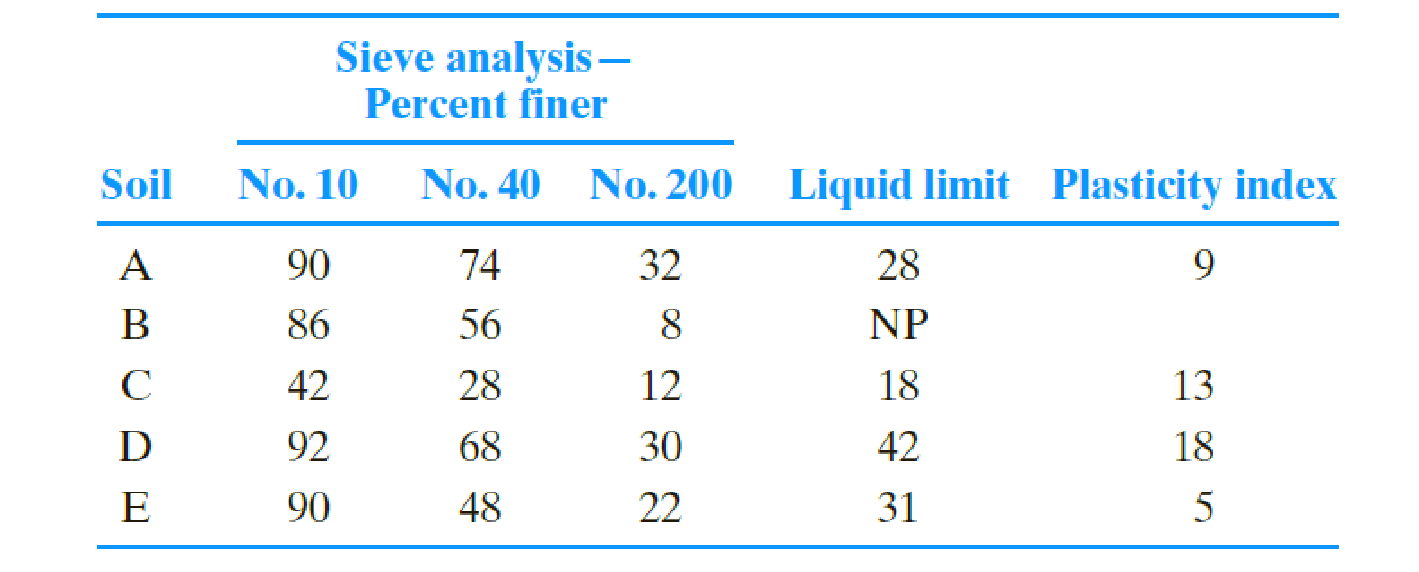Chapter 5, Problem 5.3PPrinciples of Geotechnical Enginee...

9th Edition
Braja M. Das + 1 other
ISBN: 9781305970939

Solutions

Chapter
SectionPrinciples of Geotechnical Enginee...

9th Edition
Braja M. Das + 1 other
ISBN: 9781305970939
Textbook Problem

Classify the following soils by the AASHTO classification system. Give the group index for each soil.To determine

The classification of each soil based on AASTHO classification and provides the group index for each soil.

Explanation

For soil A:

Determine the group index of soil A using the formula.

GI=(F20035)[0.2+0.005(LL40)]+0.01(F20015)(PI10)

Here, F200 is the percentage passing through No. 200 sieve, LL is the liquid limit, and PI is the plasticity index.

Refer given data.

Substitute 32 for F200, 28 for LL, and 9 for PI.

GI=(3235)[0.2+0.005(2840)]+0.01(3215)(910)=0.420.17=0.590

The above Equation yields a negative value, therefore, the group index can be taken as zero.

Thus, the group index of soil A is zero_.

Soils classified under groups A-1, A-2, and A-3 are granular materials of which 35 % or less of the particles pass through the No.200 sieve. Soils which pass more than 30 % through the No. 200 sieve are classified under the groups A-4, A-5, A-6, and A-7. These types of soils are mostly silt and clay-type materials.

Refer Table (5.1) “Classification of highways subgrade materials” in the text book.

Show the parameter specifications from Table (5.1), and parameters of the given soil as in Table 1.

 Parameter Specification in Table (5.1) Parameters of the given soil Percent passing sieve No. 10 - 90 No. 40 - 74 No. 200 35 max. 32 Plasticity index (PI) 10 max. 9

Table 1

Refer Table 1.

The soil falls under the classification of A-2-4.

Hence, the classification of the soil as per AASTHO is A-2-4(0)_ since the group index of the soil is zero.

For soil B:

For soil B, there is no plasticity, therefore, the group index of soil B is zero_.

Show the parameter specifications from Table (5.1), and parameters of the given soil as in Table 2.

 Parameter Specification in Table (5.1) Parameters of the given soil Percent passing sieve No. 10 - 86 No. 40 51 min. 56 No. 200 10 max. 8 Plasticity index (PI) NP. NP

Table 2

Refer Table 2.

The soil falls under the classification of A-3.

Hence, the classification of the soil as per AASTHO is A-3(0)_ since the group index of the soil is zero.

For soil C:

Determine the group index of soil C using the formula.

GI=(F20035)[0.2+0.005(LL40)]+0.01(F20015)(PI10)

Refer given data.

Substitute 12 for F200, 18 for LL, and 13 for PI.

GI=(1235)[0.2+0.005(1840)]+0.01(1215)(1310)=2

Still sussing out bartleby?

Check out a sample textbook solution.

See a sample solution

The Solution to Your Study Problems

Bartleby provides explanations to thousands of textbook problems written by our experts, many with advanced degrees!

Get Started

What are the potential costs of implementing a database system?

Database Systems: Design, Implementation, & Management

What is containment, and why is it part of the planning process?

Principles of Information Security (MindTap Course List)

What is an operational security control?

Management Of Information Security

A(n) ______ code stores information that can correspond to a web address or other content.

Enhanced Discovering Computers 2017 (Shelly Cashman Series) (MindTap Course List)